## The Variational Principle (Rayleigh-Ritz Approximation)

Because the ground state has the lowest possible energy, we can vary a test wavefunction, minimizing the energy, to get a good estimate of the ground state energy.for the ground state.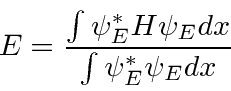For any trial wavefunction,We wish to show that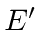errors are second order inat eigenenergies.

To do this, we will add a variable amount of an arbitrary functionto the energy eigenstate.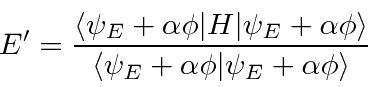Assumeis real since we do this for any arbitrary function. Now we differentiate with respect toand evaluate at zero.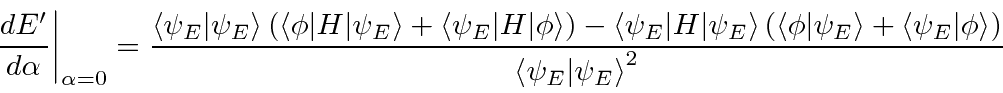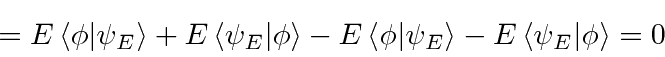We find that the derivative is zero around any eigenfunction, proving that variations of the energy are second order in variations in the wavefunction.

That is,is stationary (2nd order changes only) with respect to variation in. Conversely, it can be shown thatis only stationary for eigenfunctions. We can use the variational principle to approximately findand to find an upper bound on.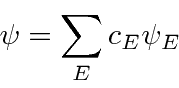For higher states this also works if trialis automatically orthogonal to all lower states due to some symmetry (Parity,...)

* Example: Energy of 1D Harmonic Oscillator using a polynomial trail wave function.*
* Example: 1D H.O. using Gaussian.*

Jim Branson 2013-04-22